大蒜废弃物负载纳米零价铁还原反应活性的定量评价研究Quantitative Evaluation of the Activity of Nano-Zero Iron Reduction Reaction on Garlic Waste

• 全文下载: PDF(1496KB)    PP.527-535   DOI: 10.12677/AEP.2018.86065
• 下载量: 276  浏览量: 1,742   国家科技经费支持

Nano-zero-valent iron has been widely used in the field of environmental pollution control due to its high efficiency of reduction and environmental friendliness. This study discusses the preparation of nano-zero-valent iron as well as the comparison of the characteristics of several nano-sized zero-valent iron preparation methods. In this study, we also aimed at the quantitative evaluation of the zero-valent iron reduction activity of nano-particles, and proposed an analytical test method based on the CrO42-/Cr3+ system. The method is sensitive and accurate in measurement and can be applied to the quantitative determination of the zero-valent iron reduction activity evaluation. On this basis, we made a comparative study of the retention ability of the prepared nano-zero-valent iron in reducing activity under a variety of storage conditions, screening and determining the appropriate storage medium, storage time and other conditions, and it was found that anhydrous ethanol can be used as a storage medium, 60% absolute ethanol + 40% deionized water for three days, the reaction time of one hour, to keep the reduction effectiveness of freshly prepared samples. Research can provide valuable reference and guidance for the preservation and transportation of nano-zero-valent iron materials. The basic data obtained in this work will be of good reference for improving the preparation, storage and application of the existing nano-zero-valent iron materials.

1. 引言

2. 实验方案和实验设计

2.1. 实验试剂及仪器

2.2. 实验方法

2.2.1. 静态吸附试验

2.2.2. 金属离子浓度检测方法

2.2.3. Cr(VI)浓度检测方法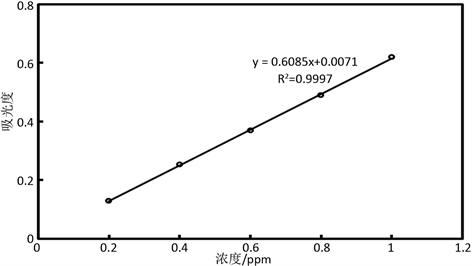Figure 1. Cr(VI) concentration determination standard curve

$y=0.608x+0.007$

2.2.4. 氧化还原电位检测方法

ORP (Oxidation-Reduction Potential)即氧化还原电位   。ORP作为介质(包括土壤、天然水、培养基等)环境条件的一个综合性指标，可用用来表征介质氧化性或还原性的相对程度。水中的每一种物质都有其特有的氧化还原特性，氧化还原电位越高，其氧化性也就越强，氧化还原电位越低，其氧化性也就越弱。电位为正表示溶液显示出氧化性，为负则说明溶液显示出还原性。ORP电极可使用于任何pH/mv测定计上，使用时可直接使用，无需标定，只有当对ORP电极品质或是测试结果有疑问时，可用ORP标准溶液检查其电位是否在200~275 mv之间，用以判断ORP电极或仪器的好坏。

2.2.5. 吸附量及吸附容量表示

$A=\frac{\left({C}_{i}-{C}_{e}\right)}{{C}_{i}}×100%$ (1)

$Q=\frac{\left({C}_{i}-{C}_{e}\right)V}{W}$ (2)

3. 负载纳米零价铁大蒜皮废弃物对Cr(VI)的还原实验结果

3.1. 初始pH的影响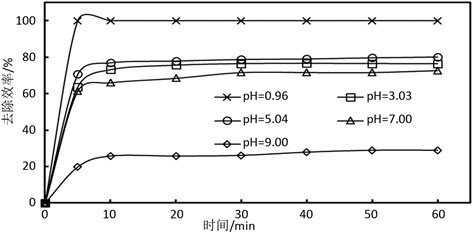Figure 2. Effect of time on the efficiency of CMGR-Fe(0) treatment of Cr(VI) under different pH conditions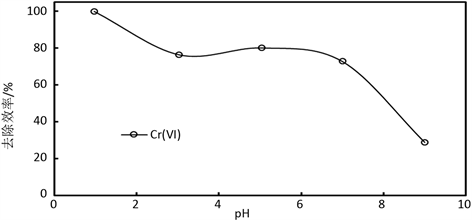Figure 3. Effect of CMGR-Fe(0) on removal efficiency of Cr(VI) at different pH

3.2. 反应机理

$\begin{array}{l}\text{Cr}\left(\text{VI}\right)+\text{GR}-{\text{Fe}}^{\left(0\right)}+{\text{H}}^{+}\to \text{Cr}\left(\text{III}\right)+\text{Fe}\left(\text{III}\right)+{\text{H}}_{2}\text{O}\\ \left(1-\text{x}\right)\text{Fe}\left(\text{III}\right)+\text{xCr}\left(\text{VI}\right)+3{\text{H}}_{2}\text{O}\to {\text{CrxFe}}_{1-x}{\left(\text{OH}\right)}_{3}+3{\text{H}}^{+}\\ \left(1-\text{x}\right)\text{Fe}\left(\text{III}\right)+\text{xCr}\left(\text{VI}\right)+2{\text{H}}_{2}\text{O}\to {\text{CrxFe}}_{1-x}\left(\text{OOH}\right)+3{\text{H}}^{+}\end{array}$Table 3. Changes in pH before and after removal of Cr(VI)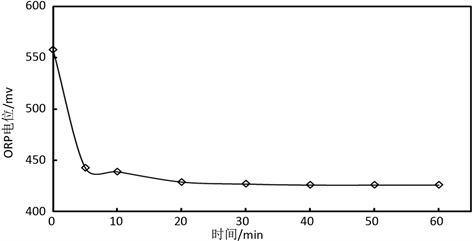Figure 4. ORP value of the system as a function of time when the pH of the solution is 1

3.3. 吸附剂量的影响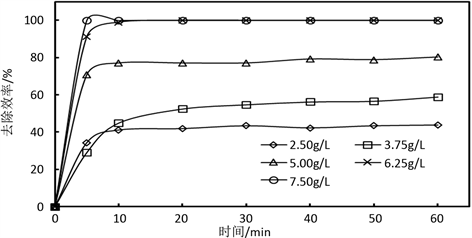Figure 5. Effect of time with different CMGR-Fe(0) addition on Cr(VI) removal efficiency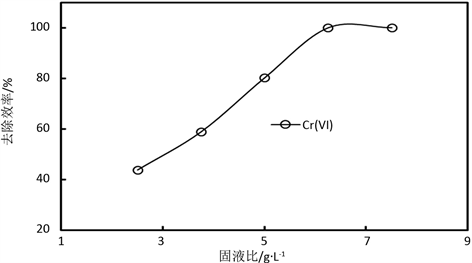Figure 6. Removal of Cr(VI) by different CMGR-Fe(0) additions

3.4. 温度的影响

4. 结论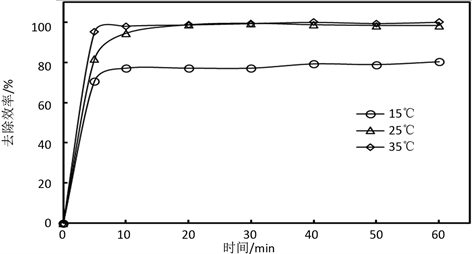Figure 7. Effect of time at different temperatures on the efficiency of CMGR-Fe(0) removal of Cr(VI)

  吉祖峰, 崔世海. 纳米零价铁的制备及其对2,4-二氯酚的降解研究[C]//全国环境化学学术大会. 上海: 中国化学会, 2011: 226.  冯丽, 葛小鹏, 王东升, 等. pH值对纳米零价铁吸附降解2,4-二氯苯酚的影响[J]. 环境科学, 2012, 1(1): 94-103.  金晓英, 李任超, 陈祖亮. 纳米零价铁活化过硫酸钠降解2,4-二氯苯酚[J]. 环境化学, 2014(5): 812-818.  Vodyanitskii, Y.N., Mineev, V.G. and Shoba, S.A. (2014) Role of Zero-Valent Iron in the Degradation of Organochlorine Substances in Ground-water. Moscow University Soil Science Bulletin, 69, 175-183. https://doi.org/10.3103/S0147687414040085  Hou, M.F., Wan, H.F., Zhou, Q.X., et al. (2009) The Dechlorination of Pen-tachlorophenol by Zerovalent Iron in Presence of Carboxylic Acids. Bulletin of Environmental Contamination & Toxicology, 82, 137. https://doi.org/10.1007/s00128-008-9574-7  辛梓弘, 凡小梅, 倪海晨, 等. 纳米零价铁的制备及其对废水中铬的去除作用研究[J]. 广州化工, 2017, 45(11): 74-76.  张鑫. 纳米零价铁去除水中重金属离子的研究进展[J]. 化学研究, 2010, 21(3): 97-100.  Matheson, L.J. and Tratnyek, P.G. (1994) Reductive Dehalogenation of Chlorinated Methanes by Iron Metal. Environmental Science & Technology, 28, 2045-2053. https://doi.org/10.1021/es00061a012  庞龙, 周庆祥, 苏现伐. 纳米零价铁修饰技术研究进展[J]. 化工进展, 2011(6): 1361-1368.  代莹, 邹金龙, 王伟, 等. 纳米零价铁去除水中污染物的研究进展[J]. 黑龙江工程学院学报, 2015(2): 46-51.  赵丹. 纳米零价铁去除水中六价铬, 甲基橙染料和三氯乙烯的研究[D]: [硕士学位论文]. 北京: 中国地质大学(北京), 2014: 46-51.  薛嵩. 生物炭负载纳米零价铁对有机污染物的去除研究[D]: [硕士学位论文]. 苏州: 苏州科技学院, 苏州科技大学, 2015.  邱月峰. 生物炭负载纳米铁镍双金属原位修复模拟地下水中三氯乙烷[D]: [硕士学位论文]. 上海: 华东理工大学, 2016.  王由好, 张永祥, 段智隆, 等. 改性沸石负载纳米铁/镍去除地下水中2,4-二氯苯酚的渗透反应格栅研究[J]. 应用化工, 2016, 45(9): 1606-1610.  冯丽. 纳米零价金属铁, 镍对2,4-二氯苯酚降解性能研究[D]: [硕士学位论文]. 北京: 中国科学院研究生院, 2011.  吴丽梅, 吕国诚, 廖立兵. 活性炭负载纳米零价铁去除污水中六价铬的研究[J]. 矿物学报, 2012(s1): 181-182.  Zhang, W., Li, X. and Cao, J. (2009) Reply to “Comments on ‘Stoichiometry of Cr(VI) Immobilization Using Nanoscale Zerovalent Iron (nZVI): A Study with High-Resolution X-Ray Photoelectron Spectroscopy (HR-XPS)’”. Industrial & Engineering Chemistry Research, 47, 2131-2139. https://doi.org/10.1021/ie8016434  杨麒, 伍秀琼, 钟宇, 等. 活性炭负载纳米零价铁去除溴酸盐的研究[J]. 湖南大学学报(自科版), 2013, 40(12): 97-102.  孙艳秋, 柳听义, 燕志芳, 等. 纳米铁的制备及其去除污染物效果的研究进展[J]. 能源环境保护, 2016, 30(3): 1-5.  互联网. 关于氧化还原电位(ORP、Eh)去极化测定法的二十个问题[J]. 互联网.  苏高强. 有机废水处理工程中的氧化还原电位的特性研究[D]: [硕士学位论文]. 郑州: 郑州大学, 2009.